• python矩阵相乘 1.点乘 import numpy as np x = np.array([[3,4],[2,16]]) b = np.linalg.inv(x) x = np.dot(x, b) print(x) result c [[1. 0.] [0. 1.]] 横乘竖 2.×乘 c import numpy as np x = np...
1.点乘
import numpy as np

x = np.array([[3,4],[2,16]])
b = np.linalg.inv(x)
x = np.dot(x, b)
print(x)

result

c
[[1. 0.]
[0. 1.]]


横乘竖

2.×乘

c
import numpy as np

x = np.array([[3,4],[2,16]])
b = np.linalg.inv(x)
x = np.multiply(x, b)
print(x)


result
[[ 1.2 -0.4]
[-0.1  1.2]]




展开全文• c=[] t=[] for i in range(len(m)): for j in range(len(n)): sum=0 for k in range(len(n)): sum+=m[i][k]*n[j][k] t.append(sum) c.append(t) t=[] print(c)
c=[]
t=[]
for i in range(len(m)):
for j in range(len(n)):
sum=0
for k in range(len(n)):
sum+=m[i][k]*n[j][k]
t.append(sum)
c.append(t)
t=[]
print(c)


展开全文• CUDA PYTHON 矩阵相乘一、CUDA线程索引二、CUDA矩阵计算1、卷积计算2、矩阵相乘三、CUDA共享内存四、CUDA python 矩阵相乘代码实践参考内容 一、CUDA线程索引 线程索引即如何根据线程层次中的blockId，gridId以及...
CUDA PYTHON 矩阵相乘一、CUDA线程索引二、CUDA矩阵计算1、卷积计算2、矩阵相乘三、CUDA共享内存四、CUDA python 矩阵相乘代码实践参考内容
一、CUDA线程索引
线程索引即如何根据线程层次中的blockId，gridId以及threadId确定线程的确切下标。可以把线程确切的下标理解为一个唯一的线程索引表示，它和线程层次中的每一个线程都是一一对应的。线程层次参看前一篇文章 CUDA PYTHON并行计算基础。例如下方图示所给出的计算方法。
对于一维的情况，如果一个Block有8个Thread，则第3个Block（下标为2，因为从0开始）的第5个Thread的确切下标就是8 x 2
+5 = 21：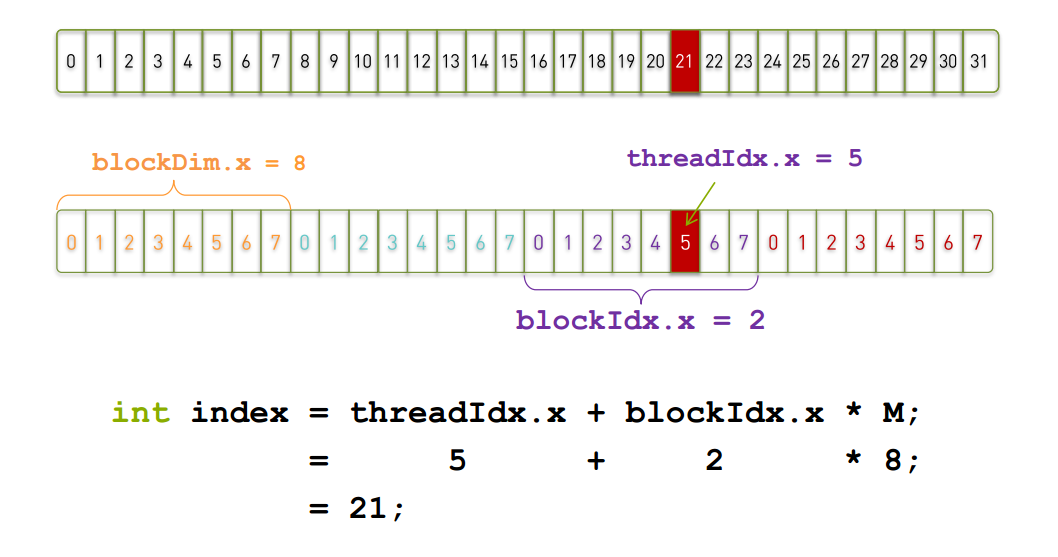如果是针对二维的情况，计算将要复杂一些，索引分为x方向和y方向两个下标。下图中的一个Grid在x方向维度大小为3，在y方向维度大小为2；一个Block在x方向维度大小为4，在y方向维度大小为3。Thread_x与Thread_y分别表示为线程在x方向和y方向的索引。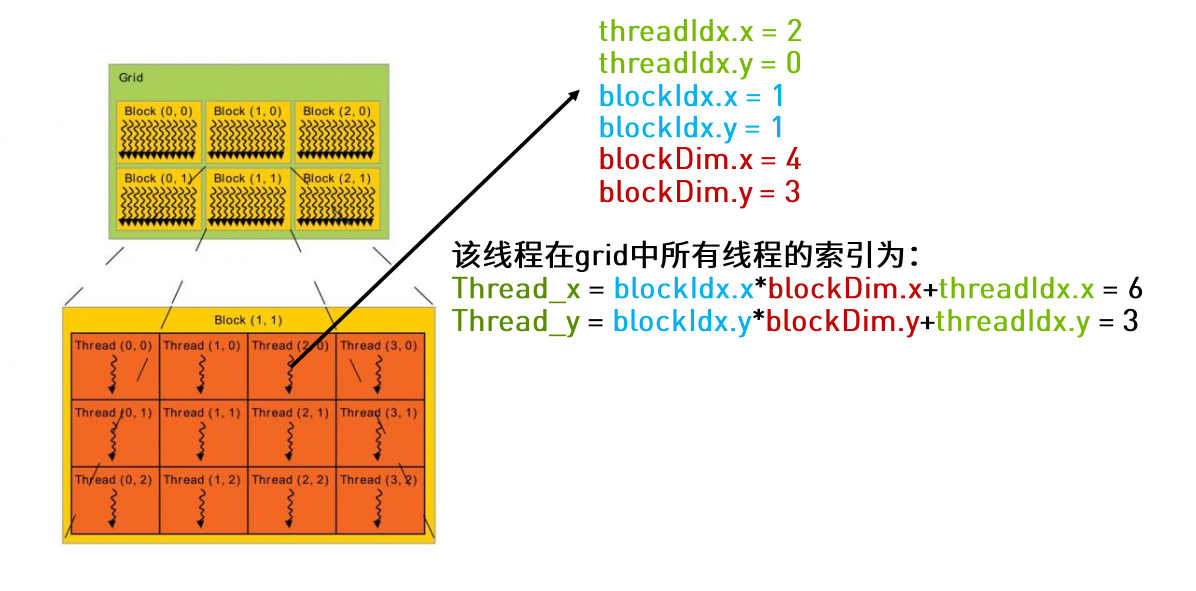如果将上方的层次模型进行展开如下图所示，将会对计算公式有一个更清晰的认识。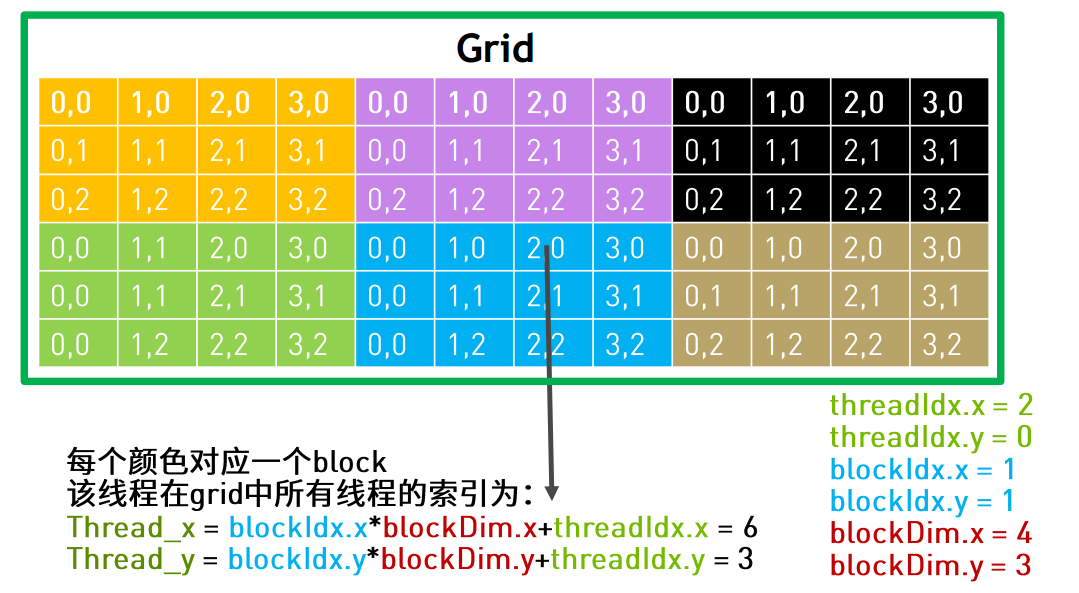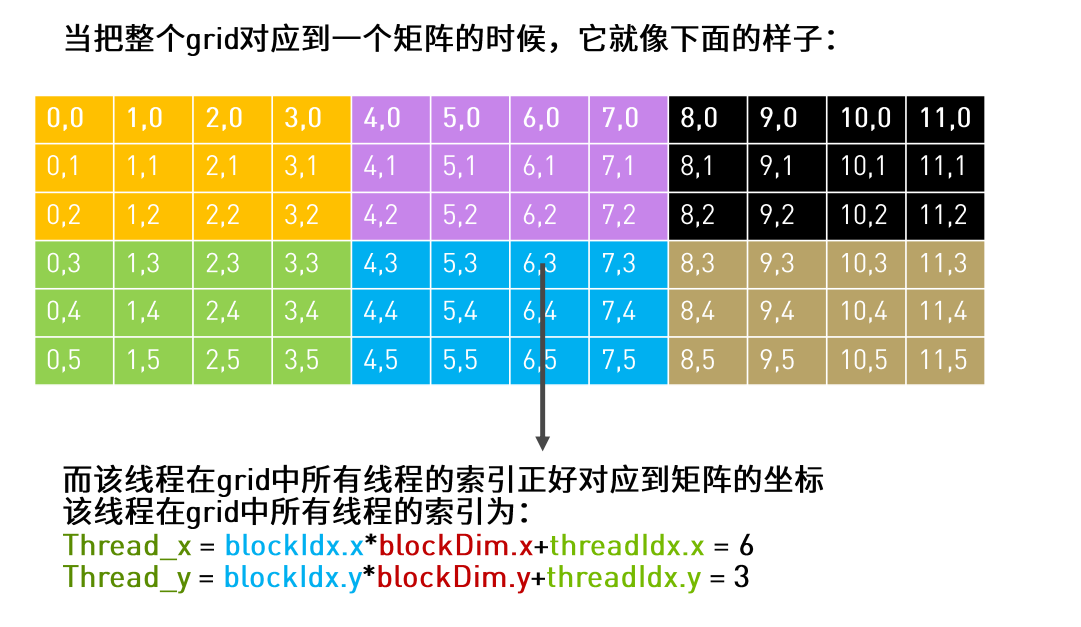如果需要处理的任务数量超过了设备所拥有和定义的线程数量（一般来说对于一个GPU设备只有一个Grid），可以采用Grid跨步（grid-stride loop） 的方法处理，即将需要处理的任务划分为多个子任务，使单个子任务能够被一个grid处理。Grid会遍历整个任务的划分依次进行执行，直到计算完所有的任务。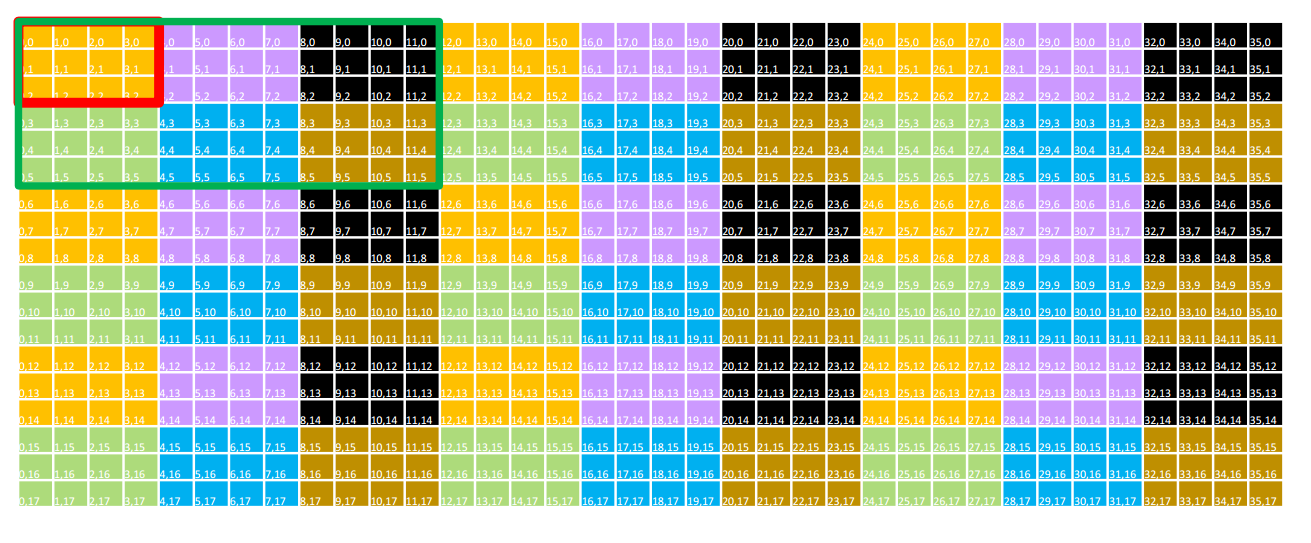如上图，绿色的框线内为一个Grid，红色框线内为一个Block，一个单元格为一个线程任务。图中单元格的颜色相同表示这些任务将会被同一个Block中的线程执行，通过上图不难看出每个Block内的每个线程将会执行9个任务。
具体的代码实现如下，stride_x和stide_y定义了跨步的步长，通过下方代码可以将任务划分到不同的线程中去执行。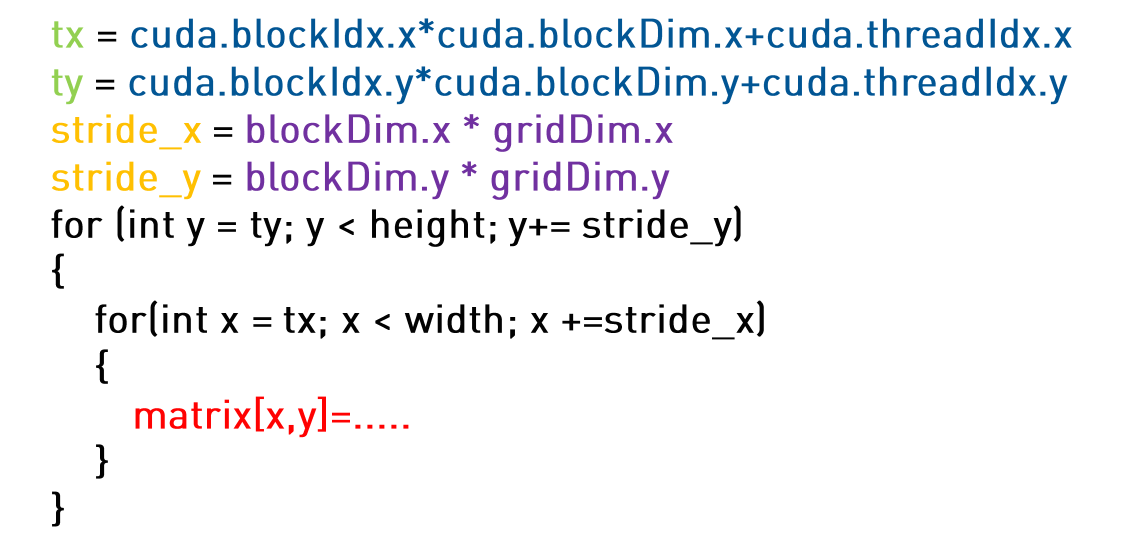二、CUDA矩阵计算
1、卷积计算
我们知道卷积计算的底层本质上还是矩阵计算，通过定义卷积核，并在图片上滑动的进行矩阵运算，可以进行图片特征的提取。一个动图展示其计算过程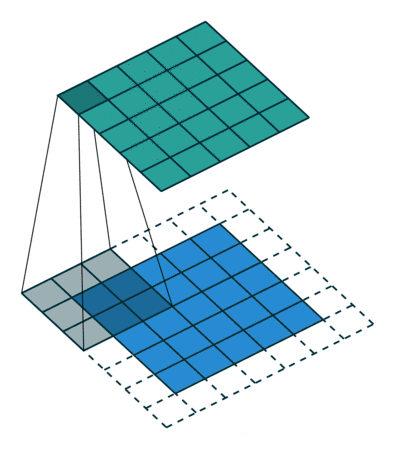在一张图片的RGB模型表示中，颜色分为红绿蓝三通道，卷积操作时针对三通道应用不同的卷积核进行特征提取操作。因为GPU设备处理单元多，可以将这些通道和卷积核进行合理的拼接，将多个通道的卷积计算合并成大的两个矩阵相乘，这一思想即使用空间换取时间，使得更多的GPU计算单元能够同时进行卷积计算任务的处理。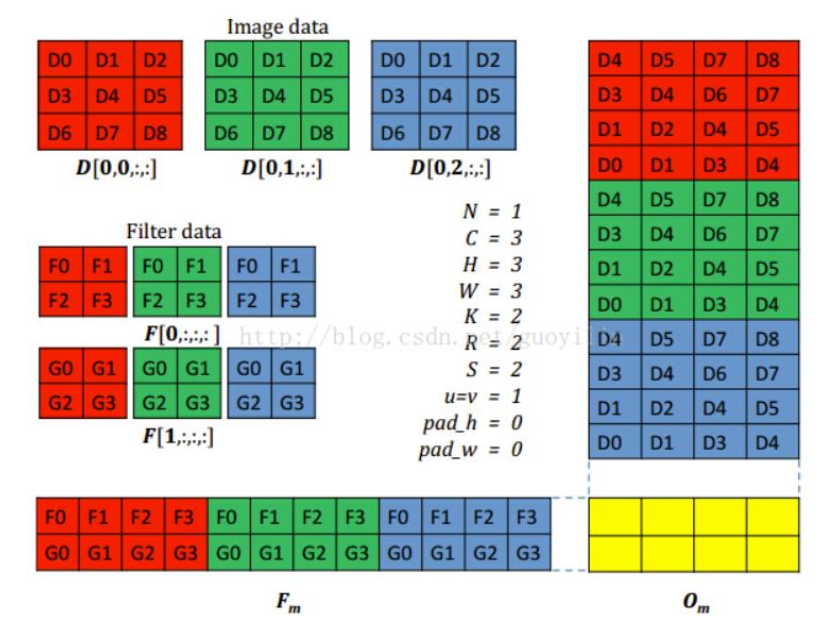2、矩阵相乘
知道了卷积计算的本质以及如何进行计算过程的转化，那么下面重点讨论如何使用GPU进行矩阵相乘任务。
线性代数中都讲到，两个矩阵M,N相乘的结果P，为M的对应行与N的对应列中的元素对应相乘再相加，得到P中对应某以元素的结果。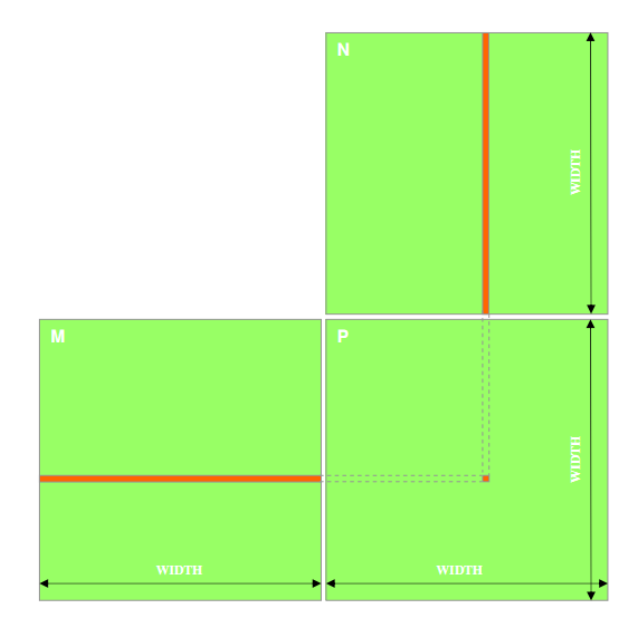在CPU上进行矩阵的乘法运算，通常需要遍历最终结果P的行数乘列数次，并且每一次中又嵌套了某一行一列的相乘相加操作。
故一般来说矩阵的乘法时间复杂度为O（n3），是一项耗时的操作。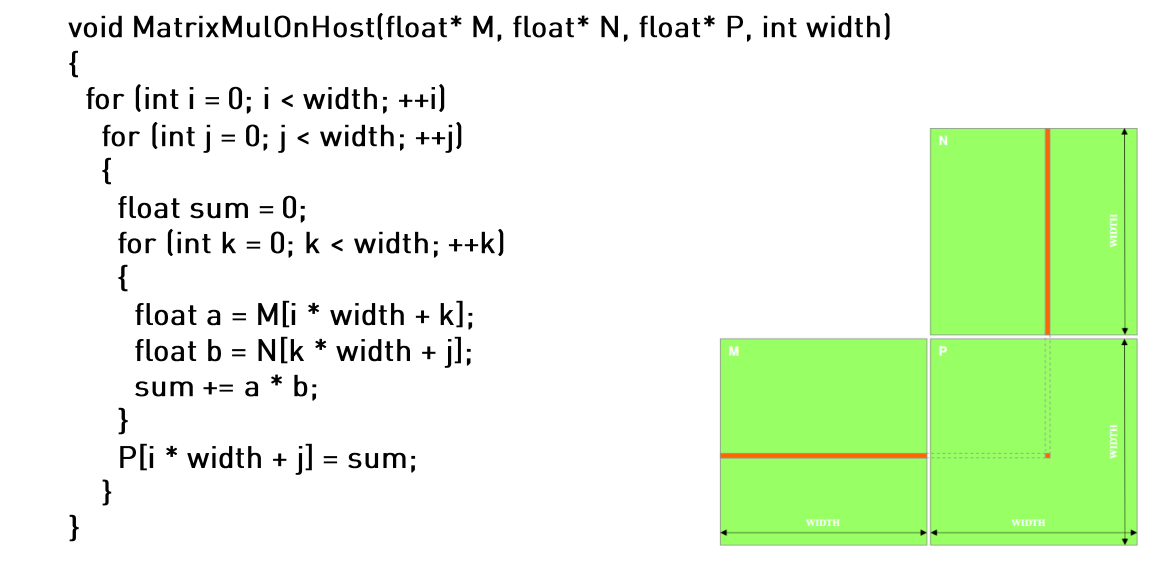我们知道，矩阵相乘的每一个一行乘以一列的操作，都是相互独立互不干扰的，因此矩阵乘法计算其实可以拆分为若干行乘列的子任务相加求和，最后拼接成一个完成的结果。这一计算要求正和CUDA编程层次类似，因此可以将行列遍历的任务按照线程层次根据不同的grid和Block划分到不同的线程中，省去了CPU计算中的最外层两个循环，应用空间换时间的思想，加大了任务处理的并行度。
利用cuda进行矩阵乘法的计算，只有累计相乘相加中用到了一层循环。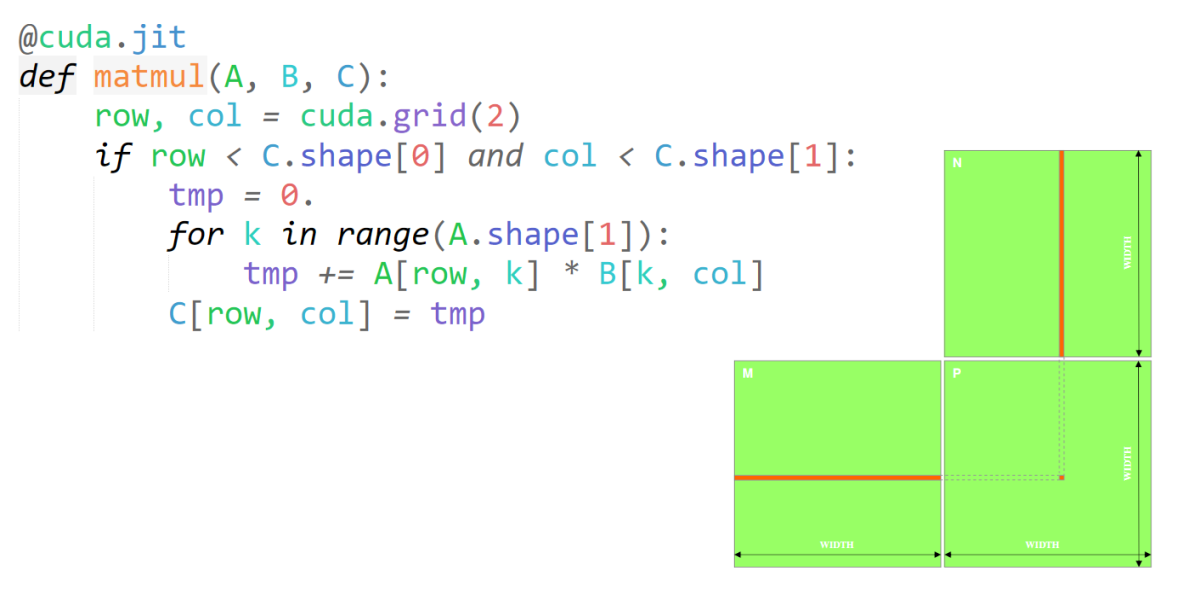上述代码中，一个Block负责计算该矩阵相乘的一个子集结果，一个线程中执行的任务即是从做左矩阵M中取一行与右矩阵N中取一列做相乘相加并设置结果集中对应元素的结果。所有的Block完成计算，其所有子集的结果拼接就是矩阵相乘的最后结果。
三、CUDA共享内存
矩阵相乘从CPU到GPU已经很大的提升了并行度，在数据规模较大时二者将产生很大的性能差距。但是GPU进行矩阵计算仍然拥有改进空间，即从内存入手。从上一篇《CUDA PYTHON 并行计算基础》中已经提到过GPU设备的内存又分为global memory（全局内存）和（shared memory）共享内存。如果没有做内存优化，数据默认都是存储在global memory中的。而我们在做矩阵相乘时，每一次都要去global memory中读取数据，会增大时间开销，如果能够让计算数据加载到shared memory，离GPU的运算单元更近一些，就能提升程序运行的性能。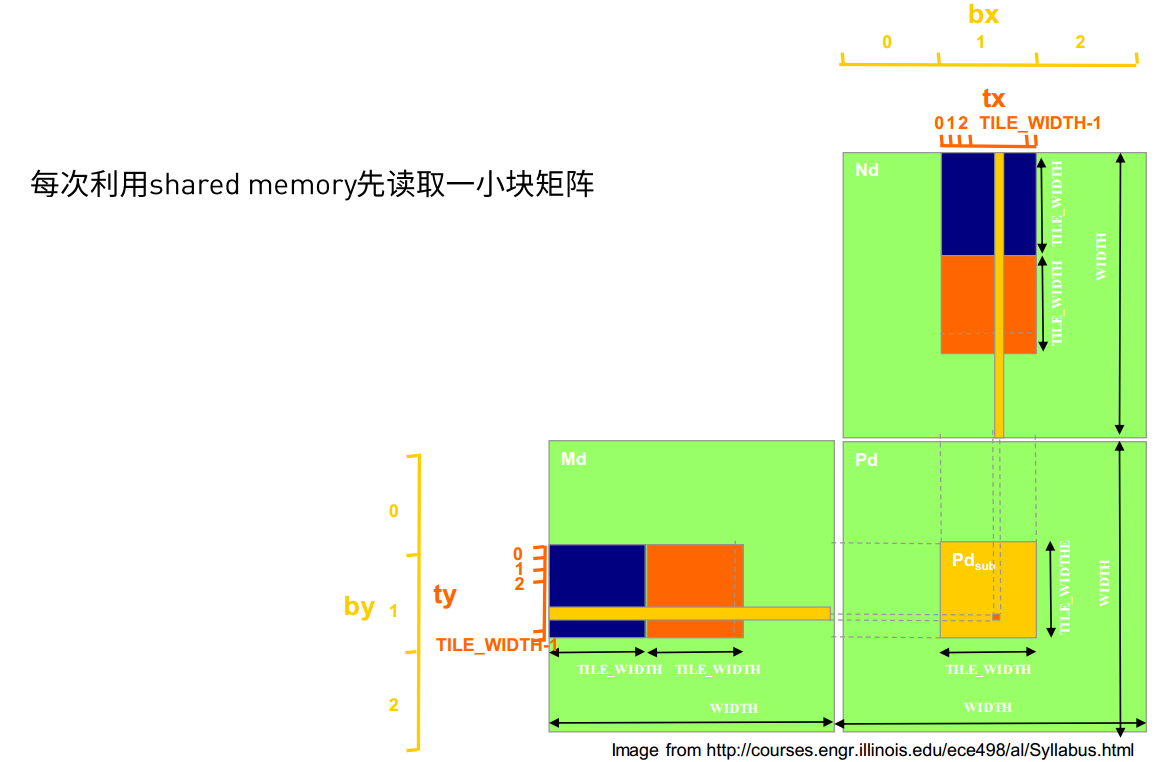通过将原来M与N矩阵中的某一块需要计算的数据提前缓存到共享内存中，计算时便不会每一次都到全局内存中去加载数据，提高计算效率。
四、CUDA python 矩阵相乘代码实践
环境要求：

python3.6+
numba
cudatoolkit

代码：
#!/usr/bin/env python
# -*- coding:utf-8 -*-
from numba import cuda, float32
import numba
import numpy
import math
import time

TPB = 16

@numba.jit(nopython=True)
def matmul_cpu(A, B, C):
for y in range(B.shape):
for x in range(A.shape):
tmp = 0.
for k in range(A.shape):
tmp += A[x, k] * B[k, y]
C[x, y] = tmp

@cuda.jit()
def matmul_gpu(A, B, C):
row, col = cuda.grid(2)
if row < C.shape and col < C.shape:
tmp = 0.
for k in range(A.shape):
tmp += A[row, k] * B[k, col]
C[row, col] = tmp

@cuda.jit()
def matmul_shared_mem(A, B, C):
sA = cuda.shared.array((TPB, TPB), dtype=float32)
sB = cuda.shared.array((TPB, TPB), dtype=float32)
x, y = cuda.grid(2)

tx = cuda.threadIdx.x
ty = cuda.threadIdx.y
if x >= C.shape and y >= C.shape:
return
tmp = 0.
for i in range(int(A.shape / TPB)):
sA[tx, ty] = A[x, ty + i * TPB]
sB[tx, ty] = B[tx + i * TPB, y]
cuda.syncthreads()
for j in range(TPB):
tmp += sA[tx, j] * sB[j, ty]
cuda.syncthreads()
C[x, y] = tmp

A = numpy.full((TPB * 130, TPB * 130), 3, numpy.float)
B = numpy.full((TPB * 130, TPB * 130), 4, numpy.float)
C_cpu = numpy.full((A.shape, B.shape), 0, numpy.float)

print("start processing in CPU")
start_cpu = time.time()
matmul_cpu(A, B, C_cpu)
end_cpu = time.time()
time_cpu = end_cpu - start_cpu
print("CPU time: " + str(time_cpu))

# start in GPU
A_global_mem = cuda.to_device(A)
B_global_mem = cuda.to_device(B)

C_global_mem = cuda.device_array((A.shape, B.shape))
C_shared_mem = cuda.device_array((A.shape, B.shape))

threads_per_block = (TPB, TPB)
blocks_per_grid_x = int(math.ceil(A.shape / threads_per_block))
blocks_per_grid_y = int(math.ceil(B.shape / threads_per_block))
blocks_per_grid = (blocks_per_grid_x, blocks_per_grid_y)

print("start processing in GPU")
start_gpu = time.time()
matmul_gpu[blocks_per_grid, threads_per_block](A_global_mem, B_global_mem, C_global_mem)
cuda.synchronize()
end_gpu = time.time()
time_gpu = end_gpu - start_gpu
print("GPU time(Global memory):" + str(time_gpu))
C_global_gpu = C_global_mem.copy_to_host()

start_gpu_shared_memory = time.time()
matmul_shared_mem[blocks_per_grid, threads_per_block](A_global_mem, B_global_mem, C_shared_mem)
cuda.synchronize()
end_gpu_shared_memory = time.time()

time_gpu_shared = end_gpu_shared_memory - start_gpu_shared_memory
print("GPU time(shared memory):" + str(time_gpu_shared))
C_shared_gpu = C_global_mem.copy_to_host()


结果展示：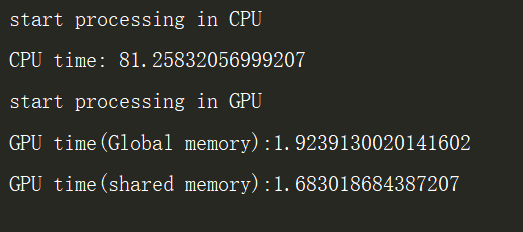可见GPU加速后的矩阵计算已在速度上遥遥领先，经过共享内存加速的程序也提升了计算性能。基于笔者采用的时GTX 860 4G显存的笔记本GPU设备做的内存加速，所以效果并非特别明显。在一些具有高性能大容量显存的GPU设备上进行内存优化将会获得数倍的性能提升。
参考内容
https://blog.csdn.net/LN_IOS/article/details/80538570
https://info.nvidia.com/364565-ondemand.html
https://images.nvidia.cn/cn/webinars/2020/jun23/CUDA-python%E7%B3%BB%E5%88%97%E8%AF%BE%E7%A8%8B-%E5%B9%B6%E8%A1%8C%E8%AE%A1%E7%AE%97%E5%9F%BA%E7%A1%80%20—%E7%9F%A9%E9%98%B5%E8%AE%A1%E7%AE%97%E4%BB%A5%E5%8F%8A%E5%85%B1%E4%BA%AB%E5%86%85%E5%AD%98.pdf


展开全文机器学习
• python 矩阵相乘 np.array() 及np.mat() 【笔记】 python实现矩阵相乘的时候，矩阵通常自定义为np.array()或者np.mat()，乍一看好像打印出来都是一样 例： [[1 0 0 ] [0 1 0] [0 0 1]] 打印出来都是这个格式，但是在...
python 矩阵相乘 np.array() 及np.mat()
【笔记】
python实现矩阵相乘的时候，矩阵通常自定义为np.array()或者np.mat()，乍一看好像打印出来都是一样
例：
[[1 0 0 ]
[0 1 0]
[0 0 1]]
打印出来都是这个格式，但是在进行矩阵相乘的时候，如：
矩阵A乘以B
A*B
此时，np.array()相乘是两个数组相同位置的元素相乘，而np.mat()进行的是线代中的矩阵的乘法。
要想使得其乘法都是线代中矩阵相乘，使用np.dot(）即可。
C=np.dot(A,B）

`
展开全文• a=int(input()) c=int(input()) b=int(input()) l1=[] l2=[] l3=[[0 for ij in range(c)]for ji in range(a)] for ii in range(a): x=input() l1.append(x.split()) print(l1) for iii in range(b): ...
• # -*- coding: utf-8 -*- """ Spyder Editor This is a temporary script file. """ import torch import numpy as np x=[2,4,5,6] y=[2,4,5,6] z=[] x_t = torch.tensor([x]) ...res = torch.cat(.
• 矩阵相乘： 输入两个整数类型的矩阵mat1（m行d列）和mat2（d行n列），返回矩阵相乘后的结果mat1*mat2（m行n列）。矩阵均用二维列表进行表示。 def func3(m1, m2): m = len(m1) n = len(m2) m3 = [[0 for i ...
• ## Python计算矩阵相乘

千次阅读 2015-08-27 17:54:47
Python学习手册-第3版》中提到python可以进行复杂的数学计算，甚至堪比MATLAB，随便写了一个矩阵相乘计算的本来应该要导入numpy这个模块的，但一直在windows下没有弄好，暂时就木有导入强大的模块功能了，苦逼的...
• ## python实现矩阵相乘

万次阅读 多人点赞 2018-08-27 13:31:22
面试时，面试官让现现场用python写两个矩阵相乘的程序，不可以直接调api。十分钟以后程序写了出来，但是自己看着都别扭，首先代码冗余，这很不python，其次用的时间有点长啊，这种程序花了十分钟，估计面试官也挺...
• 今天小编就为大家分享一篇Python实现矩阵相乘的三种方法小结，具有很好的参考价值，希望对大家有所帮助。一起跟随小编过来看看吧
• /usr/bin/env python #coding=utf-8 import numpy as np def MatrixMultiply(a, b): n11,n12=np.shape(a) n21,n22=np.shape(b) n=max(n11,n22) c=np.zeros([n11,n22],dtype=int) for i ...
• 网上相关都是方阵相乘，因此看不出来python中行列具体如何表示 题目：，求A*B 代码如下: import numpy as np A=np.mat([[1,1],[2,1]]) B=np.mat([,]) print(A*B) 结果为 ...
• ## Python实现矩阵相乘

千次阅读 2015-03-20 17:40:40
/usr/bin/env python #coding=utf-8def MatrixMultiply(a, b): n=len(a) c=[*n for row in range(n)] #初始化c为n行n列的全零矩阵 for i in range(0, n): for j in range(0, n): #c[i][j]
• ## python 实现矩阵相乘

千次阅读 2015-06-12 16:01:01
优势在于，矩阵的数据组织方式适合分布式计算 # coding:utf-8 __author__ = 'taohao' import random class Matrix(object): def build_matrix_a(self, row, col): """ matrix: 1 0 2分布式计算 matrix
• 主要介绍了Python实现的矩阵转置与矩阵相乘运算,结合实例形式分析了Python针对矩阵进行转置与相乘运算的相关实现技巧与操作注意事项,需要的朋友可以参考下
• 参考张老师的mapreduce 矩阵...下面是我用python版本的mapreduce 矩阵相乘矩阵相乘的思路详见张老师的博客，对于两个矩阵m1和m2，mapreduce的计算过程如下： 这里面最主要的地方是key的构成，map输出的key是相...
• 分别实现矩阵相乘的3种算法，比较三种算法在矩阵大小分别为22∗222^2*2^2, 23∗232^3*2^3, 24∗242^4*2^4, 25∗252^5*2^5, 26∗262^6*2^6, 27∗272^7*2^7, 28∗282^8*2^8, 29∗292^9*2^9时的运行时间与MATLAB自带的...基础数学
• ## define the matrix multiply function def multiply_matrix(matrix,n): result=[] for i in range(n): rows=[] for j in range(n): column=[a[j] for a in matrix] ans=0 for m in range(n): ...
• ## 矩阵相乘（Python）

千次阅读 2019-07-08 14:35:00
• ## python中的矩阵相乘

万次阅读 2019-02-18 21:58:25
1. python中的矩阵（maxtrix）相乘与线性代数的算法一样，例如： 首先引入numpy import numpy as np; A =np.array([[1,2,3], [4,5,6]]) B = np.array([[1,4], [2,5], [3,6]]) C = A * 2 D = np.dot(A,.....
• 主要介绍了python的几种矩阵相乘的公式详解,文中通过示例代码介绍的非常详细，对大家的学习或者工作具有一定的参考学习价值,需要的朋友可以参考下...

# python矩阵相乘python 订阅